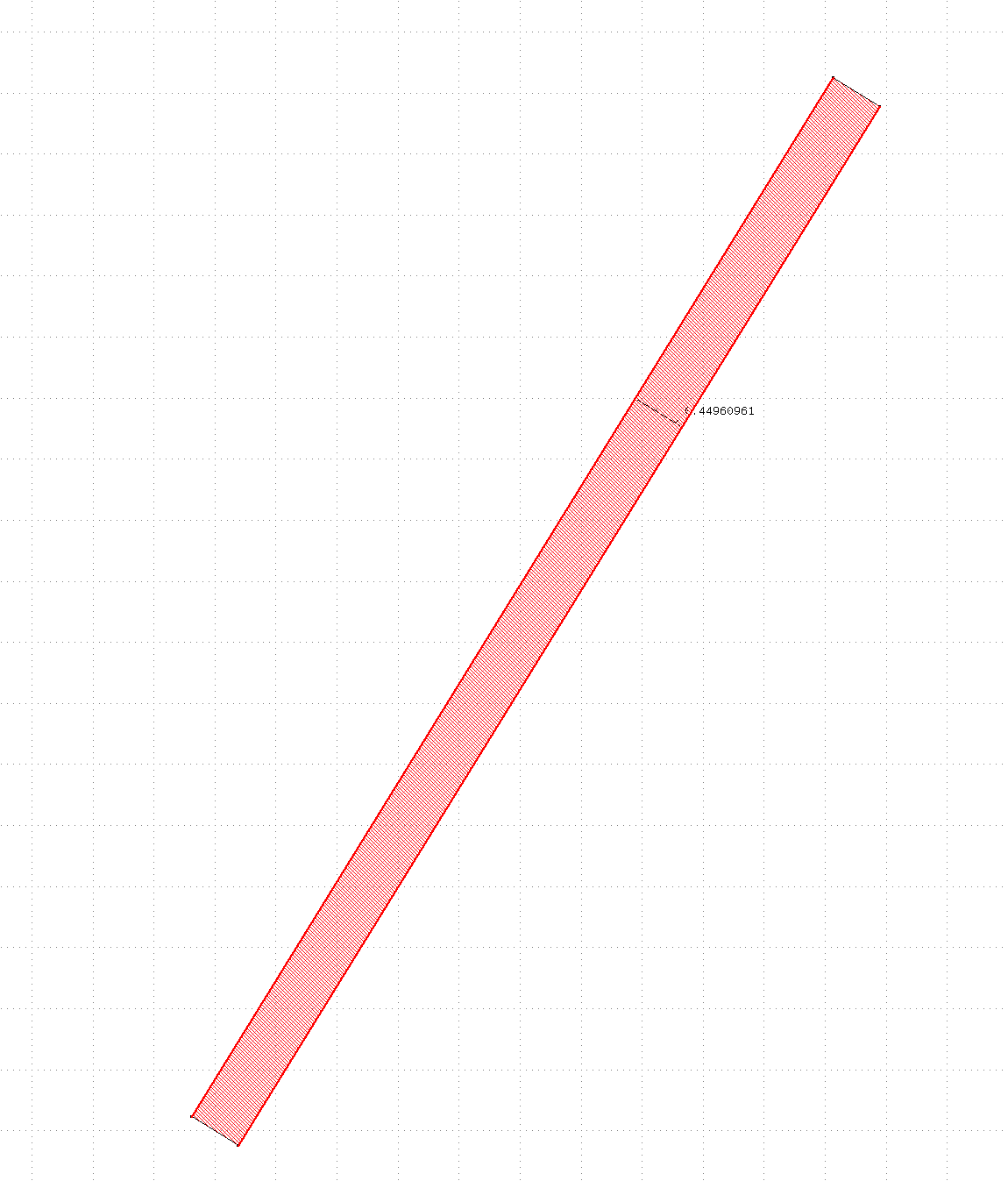# How to remove fake error when use 'width' to detect a sloped rectangle?

## I want to detect a sloped rectangle's width, so I used the code

report("DRC report")

# Drawing layers

ln_wg = input(1, 0)

# CONT_W

min_cont_w = 350.nm

# A "tolerance" applied to allow for polygon roughness:

delta = 2*2**0.5.dbu

# r_cont_w = ln_wg.width(square, min_cont_w - delta)

r_cont_w = ln_wg.raw.width(projection, min_cont_w - delta, angle_limit(89.5))

## r_cont_w.output("CONT_W: contact width < #{'%.12g' % min_cont_w} µm")

but the above code cannot detect sloped rectangle, the fake error is that whatever the angle is, there will exist an error in the edge pair, even if the width is satisfied. The output is show in the following figure, Could anyone help me a little bit? Thanks.